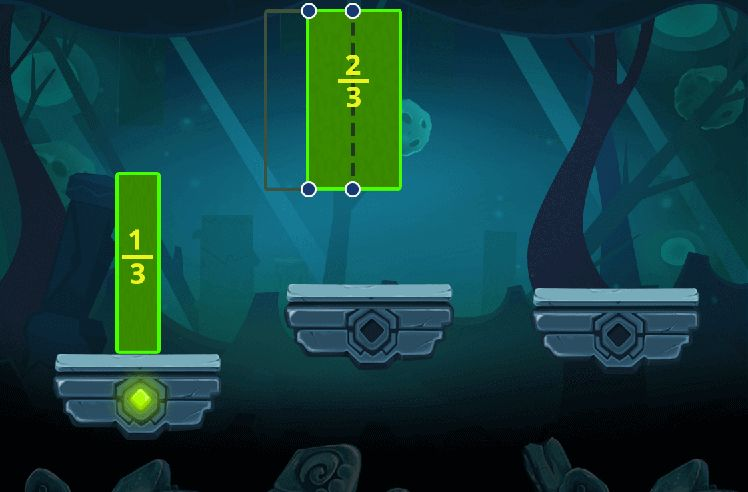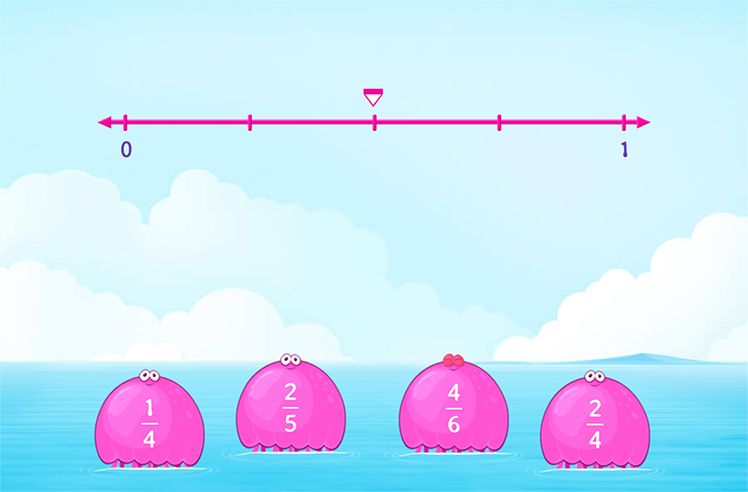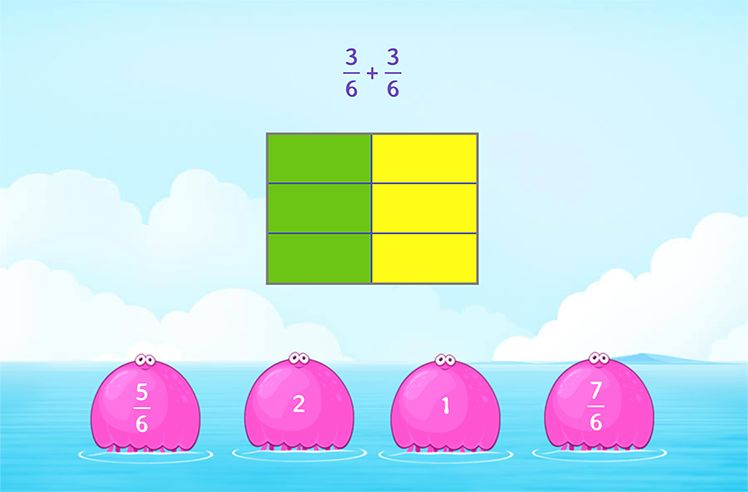# Natural Numbers – Definition with Examples

» Natural Numbers – Definition with Examples

## Definition of Natural Numbers

Natural numbers are all positive integers from 1 to infinity. They are also called counting numbers as they are used to count objects. Natural numbers do not include 0 or negative numbers.

We need numbers in our everyday life be it for counting objects, telling time, numbering houses etc. Numbers that help us in counting and representing quantities are called natural numbers. These include 1, 2, 3, 4, 5, 6 and go on till infinity.

Here we can see that 1 is the smallest natural number and each subsequent natural number is exactly one more than the previous one. Thus, the numbers that come in between these numbers are not natural numbers like fractions, decimals etc.

## History of Natural Numbers

It is presumed that natural numbers originated from the words used for counting objects, which begin with one. A place value system for numerals 1 (one) and 10 (ten) were first developed by Babylonians.

## Types of Natural Numbers

1. Odd natural numbers

The odd natural numbers are the positive numbers that are not divisible by 2.

For example : 29, 677, 89901 etc.

1. Even natural numbers

The even natural numbers are the positive numbers that are divisible by 2.

For example: 28, 456, 6022 etc.

## Properties of Natural Numbers

Here are some of the significant properties of natural numbers.

• Closure Property
• Commutative Property
• Associative Property
• Distributive Property
1. Closure Property of addition and multiplication.

When you add or multiply two natural numbers, the result will always be a natural number.

• Examples of closure property of addition: 2 + 2 = 4, 3 + 4 = 7, 5 + 5 = 10

In each case, the result of the addition of natural numbers is a natural number.

• Examples of closure property of multiplication: 2 × 2 = 4, 3 × 2 = 6, 5 × 5 = 25

In each case, the result of the multiplication of natural numbers is a natural number.

However, in the case of division and subtraction, this property does not hold. Subtracting or dividing two natural numbers will not always yield a natural number.

• Examples of subtraction: 4 – 6 = –2, 5 – 3 = 2, 6 – 9 = –3

The second case resulted in a natural number but the first and third ones did not.

• Examples of division: 10 ÷ 3 = 3.33, 9 ÷ 3 = 3, 15 ÷ 4 = 3.75

The first and third cases did not result in natural numbers.

1. Associative Property of addition and multiplication.

The sum or product of natural numbers remains unchanged even if the grouping of numbers is changed. However, this does not hold for division and subtraction.

• Examples of associative property of addition: 2 + (5 + 6) = 13 and (2 + 5) + 6 = 13
• Examples of associative property of multiplication: 2 × (3 × 4) = 24 and (2 × 3) × 4 = 24

Let us now look at the nature of subtraction and division, considering this property.

• Examples of subtraction: 4 – (10 – 2) = –4 and (4 – 10) – 2 = –8
• Examples of division: 5 ÷ (6 ÷ 3) = 2.5 and (5 ÷ 6) ÷ 3 = 0.27
1. Commutative Property of addition and multiplication

If we change the order of natural numbers during multiplication and addition, the result does not change.

For instance,

• 6 + 5 = 11 and 5 + 6 = 11
• 2 × 4 = 8 and 4 × 2 = 8

The commutative property does not apply to subtraction and division of natural numbers.

Examples for subtraction and division:

• 5 – 3 = 2 and 3 – 5 = –2
• 6 ÷ 3 = 2 and 3 ÷ 6 = 0.5
1. Distributive Property

According to the distributive property of multiplication over addition, if we multiply the total of two addends by a number or multiply each addend individually and then add them, the result will be the same.

Example: 2 × (5 + 3) = (2 x 5) + (2 x 3) = 16

This property also holds true in the case of multiplication over subtraction.

Example: 2 x (5 – 3) = (2 x 5) – (2 x 3) = 4

## Fun Facts

• There is no largest natural number.
• By simply adding 1 to the current natural number, you will get another natural number.
• Natural numbers go on forever.

## Solved Examples

Let us better understand the concept with these examples.

1. Pick natural numbers from the following list:

10, 6/2, 4.66, 22, 1564, –6.

Ans. The natural numbers are 10, 22, and 1564. Negative numbers, decimal numbers, and fractions are not considered as natural numbers.

1. List the first ten natural numbers.

Ans. The answer is 1, 2, 3, 4, 5, 6, 7, 8, 9, and 10, as the natural numbers start from 1.

1. What is the difference between any two consecutive natural numbers?

Ans. The difference between any two consecutive natural numbers is always 1.

## Practice Problems

### 1Between which two natural numbers does the fraction 18/3 lie?

10 and 12
5 and 7
7 and 9
12 and 14
CorrectIncorrect
Correct answer is: 5 and 7
Because when we divide 18 by 3, we get the number 6, which lies between 5 and 7.

### 2If m and m are two natural numbers, then:

m + n = n + m
m – n = n – m
m / n = n / m
None of these
CorrectIncorrect
Correct answer is: m + n = n + m
As the commutative property states that if we change the order of natural numbers during multiplication and addition, the result does not change. This property does not apply for subtraction and division.

### 3Which property of natural numbers holds for 2 + (5 + 6) = 13 and (2 + 5) + 6 = 13?

associative property of multiplication
associative property of subtraction
CorrectIncorrect
The associative property of addition holds for the given problem. The property states that the sum of natural numbers remains unchanged even if their grouping is varied.

### 4Which of the following examples correctly state commutative property?

6 + 5 = 11 and 5 + 6 = 11
2 + (5 + 6) = 13 and (2 + 5) + 6 = 13
2 × (5 + 3) = (2 x 5) + (2 x 3) = 16
4 – 6 = –2
CorrectIncorrect
Correct answer is: 6 + 5 = 11 and 5 + 6 = 11
As the commutative property states that changing the order of natural numbers during multiplication and addition will not change the result.

No. Zero is neither positive nor negative. Since natural numbers include all positive integers from 1 to infinity, zero is not included in the collection.

All natural numbers are whole numbers, but all whole numbers are not considered natural. 0 is the exception.

Ans. Natural numbers are used for counting objects in the most natural and instinctive manner. If you can count them on your fingers, the numbers can be deemed natural.

Conclusion

In this article, we have learned about natural numbers and their various properties. Read more about natural numbers and other interesting mathematical terms on SplashLearn, a game-based learning platform.

Related math vocabulary: Whole Numbers, Prime Numbers, Composite Numbers.Introduction to Fractions

Play NowFractions on Number Line

Play NowFractions Operations

Play Now